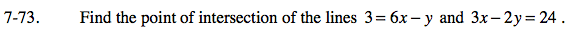### Home > AC > Chapter 7 > Lesson 7.2.3 > Problem7-73

7-73.

Find the point of intersection of the lines 3 = 6x − y and 3x − 2y = 24. Homework Help ✎One strategy to solve this problem is to solve it as a system of equations.
3x − 2y = 24
6xy = 3

Multiply the bottom equation by −2 to cancel out the y's.

3x − 2y = 24
−12x + 2y = −6
−9x = 18

Solve for x, then substitute the value back into one of the original equations to solve for y.